# Percentages + system of equations - math problems

#### Number of problems found: 81

• Together m+wWomen 30%. Men are 360 more. How many are together?
• The city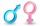In the city is 2/4 of women married to 3/4 men. What percentage of townspeople is single (not married)?
• ParticipantsOf the 48 participants, six women are fewer than men and 24 children less than all adults. Calculate what percentage of all participants on tour are children.
• EmployeesThere are 1116 people working in three factory halls. In the first one, there are 18% more than the third, and 60 persons more than the second. How many employees work in individual halls?
• ContestantsThe three best contestants are to divide the total prize of CZK 4,200. The second gets 20% more than the third. And the first one gets 200 CZK less than the second and the third together. How much will everyone get?
• Two workers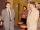Two workers make 138 parts/items. The first one produces 30% more than the second. How many items will each produce?
• Suit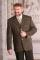The suit cost 119 euros. How much cost the pants if it is 50% cheaper than the jacket.
• Crowns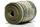1180 crowns is divided into three people that A got 20% less than B and C by 15% more than B. How many got A,B and C?
• Comparing salariesSpouses compared salaries. Mrs. Janka found that a 20% higher salary than Mr Jaroslav. Together earned € 1,430. How much they earn each of them?
• Enterprise company30% of all employees in the enterprise are women. Men are 360 more. How many employees are in the company?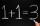Number 839 divide into the two addends that the first was 17 greater than 60% of the second. Determine these addends.
• Company and employeesThere are 370 employees in the company - women are 15% less than men. How many men work in the company?
• Container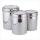First day got off 1/4 water from full container, second day 1/5 of the remaining water. How many percent remain container filled?
• ClassroomThere are eighty more girls in the class than boys. Boys are 40 percent and girls are 60 percent. How many are boys and how many girls?
• Unknown number 10Number first increased by 30%, then by 1/5. What percentage we've increased the original number?
• RepairingThree employees earned a total of € 469 for repairing the equipment. They split so that the first got 20% more than the second, and the third 15% more than the second. How many euros did everyone get?
• In the classroomThere are 30 boys and a few girls in the class. In the six months, 28 boys and all girls benefited, which was 95% of all pupils. How many pupils are there in the classroom?
• Three workers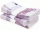The three workers received € 2,850 together for the work done. They divided them according to the time worked so that the first received 20% less than the second and the third € 50 more than the second. How much EUR did each worker receive?
• Tickets1260 tickets sold. On the first day, 80% was sold on the second day was sold. How many tickets were sold first and how much the next day?
• The hikerThe hiker will travel 40% of the route on the first day 1and/3 of the rest od second day. Last day 30 km. What was the length of the 3-day trip? How many kilometers did he walk each day?

Do you have an interesting mathematical word problem that you can't solve it? Submit a math problem, and we can try to solve it.

We will send a solution to your e-mail address. Solved examples are also published here. Please enter the e-mail correctly and check whether you don't have a full mailbox.

Please do not submit problems from current active competitions such as Mathematical Olympiad, correspondence seminars etc...

Our percentage calculator will help you quickly calculate various typical tasks with percentages. Do you have a system of equations and looking for calculator system of linear equations? Percentages Problems. System of equations - math word problems.